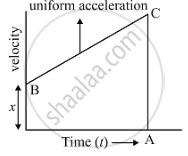# A body with an initial velocity x moves with a uniform acceleration y. Plot its velocity-time graph. - Science

Graph

A body with an initial velocity x moves with a uniform acceleration y. Plot its velocity-time graph.

#### Solution

We have to plot a graph between velocity and time. From the graph we can conclude that the curve is a straight line showing uniform acceleration.Concept: Velocity - Time Graphs
Is there an error in this question or solution?

#### APPEARS IN

Lakhmir Singh Science Class 9 Physics
Chapter 1 Motion
Hots Questions | Q 55 | Page 43